# The COUNTREG Procedure

### ZEROMODEL Statement

• ZEROMODEL dependent variable<zero-inflated regressors> </options>;

The ZEROMODEL statement is required if you specify either ZIP or ZINB in the DIST= option in the MODEL statement. If ZIP or ZINB is specified, then the ZEROMODEL statement must follow immediately after the MODEL statement. The dependent variable in the ZEROMODEL statement must be the same as the dependent variable in the MODEL statement.

The zero-inflated (ZI) regressors appear in the equation that determines the probability (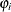) of a zero count. Each of these q variables has a parameter to be estimated in the regression. For example, let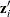be the ith observation’s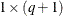vector of values of the q ZI explanatory variables (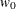is set to 1 for the intercept term). Thenis a function of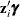, where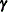is the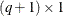vector of parameters to be estimated. (The ZI intercept is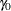; the coefficients for the q ZI covariates are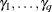.) If this option is omitted, then only the intercept termis estimated. The “Parameter Estimates” table in the displayed output gives the estimates for the ZI intercept and ZI explanatory variables; they are labeled with the prefix "Inf_". For example, the ZI intercept is labeled "Inf_intercept". If you specify Age (a variable in your data set) as a ZI explanatory variable, then the “Parameter Estimates” table labels the corresponding parameter estimate "Inf_Age".

You can specify the following options after a slash (/):

specifies the distribution function to use to compute probability of zeros. The following distribution functions are supported:

LOGISTIC

specifies the logistic distribution.

NORMAL

specifies the standard normal distribution.

If this option is omitted, then the default ZI link function is logistic.

OFFSET=variable

specifies a variable in the input data set to be used as a zero-inflated (ZI) offset variable. The ZI offset variable is included as a term, with its coefficient restricted to 1, in the equation that determines the probability () of a zero count. The ZI offset variable cannot be the response variable, the offset variable (if any), or one of the explanatory variables. The name of the data set variable that is used as the ZI offset variable is displayed in the “Model Fit Summary” output, where it is labeled as "Inf_offset".

SELECT=INFO<(option)>
SELECTVAR=INFO<(option)>

specifies variable selection.

SELECT= INFO requests that the variable selection method be based on an information criterion. For more information, see the section Variable Selection Using an Information Criterion. You can specify the following options:

DIRECTION=FORWARD | BACKWARD

specifies the search algorithm to use in the variable selection method. By default, DIRECTION=FORWARD.

CRITER=AIC | SBC

specifies the information criterion to use in the variable selection. By default, CRITER=SBC.

MAXSTEPS=value

specifies the maximum number of steps to allow in the search algorithm. The default is infinite; that is, the algorithm does not stop until the stopping criterion is satisfied.

LSTOP=value

specifies the stopping criterion. The value represents the percentage of decrease or increase in the AIC or SBC that is required for the algorithm to proceed; it must be a positive number less than 1. By default, LSTOP=0.

RETAIN(variable1 <variable2...>)

requests that the variables named within the parentheses be retained during the variable selection process.